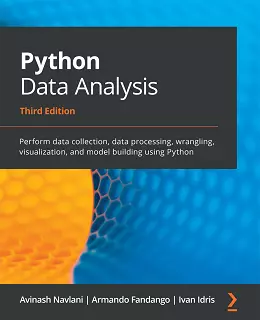# Python Data Analysis – Third Edition### eBook Details:

• Paperback: 478 pages
• Publisher: WOW! eBook; 3rd edition (February 5, 2021)
• Language: English
• ISBN-10: 1789955246
• ISBN-13: 978-1789955248

### eBook Description:

Python Data Analysis, 3rd Edition: Understand data analysis pipelines using machine learning algorithms and techniques with this practical guide

Data analysis enables you to generate value from small and big data by discovering new patterns and trends, and Python is one of the most popular tools for analyzing a wide variety of data. With this book, you’ll get up and running using Python for data analysis by exploring the different phases and methodologies used in data analysis and learning how to use modern libraries from the Python ecosystem to create efficient data pipelines.

Starting with the essential statistical and data analysis fundamentals using Python, you’ll perform complex data analysis and modeling, data manipulation, data cleaning, and data visualization using easy-to-follow examples. You’ll then understand how to conduct time series analysis and signal processing using ARMA models. As you advance, you’ll get to grips with smart processing and data analytics using machine learning algorithms such as regression, classification, Principal Component Analysis (PCA), and clustering. In the concluding chapters, you’ll work on real-world examples to analyze textual and image data using natural language processing (NLP) and image analytics techniques, respectively. Finally, the Python Data Analysis, Third Edition book will demonstrate parallel computing using Dask.

• Explore data science and its various process models
• Perform data manipulation using NumPy and pandas for aggregating, cleaning, and handling missing values
• Create interactive visualizations using Matplotlib, Seaborn, and Bokeh
• Retrieve, process, and store data in a wide range of formats
• Understand data preprocessing and feature engineering using pandas and scikit-learn
• Perform time series analysis and signal processing using sunspot cycle data
• Analyze textual data and image data to perform advanced analysis
• Get up to speed with parallel computing using Dask

By the end of this Python Data Analysis, 3rd Edition book, you’ll be equipped with the skills you need to prepare data for analysis and create meaningful data visualizations for forecasting values from data.